## 函数结构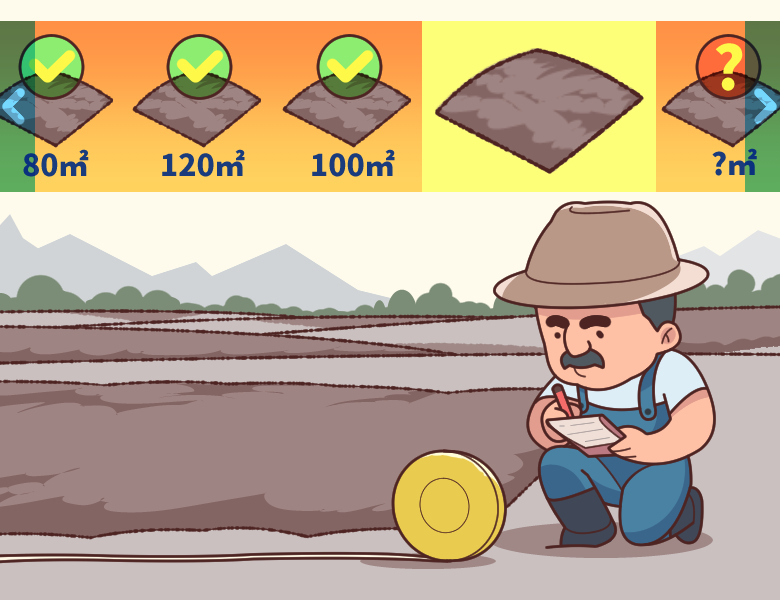``````def getArea(length):
area = length*length
print(area)

getArea(13)
getArea(7)``````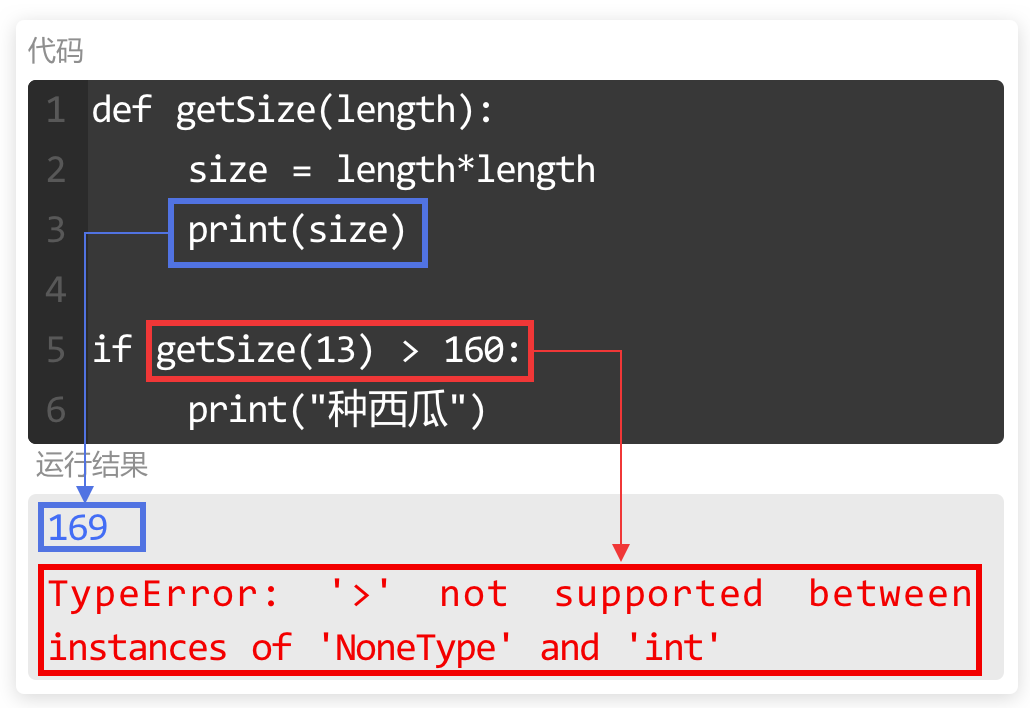## 函数返回值

``````def getArea(length):
area = length*length
return area

result = getArea(13)
print(result)``````

return

`return` 位置一般都是在函数的末尾，这样才能停止函数内的代码运行并将 `return` 后的值返回。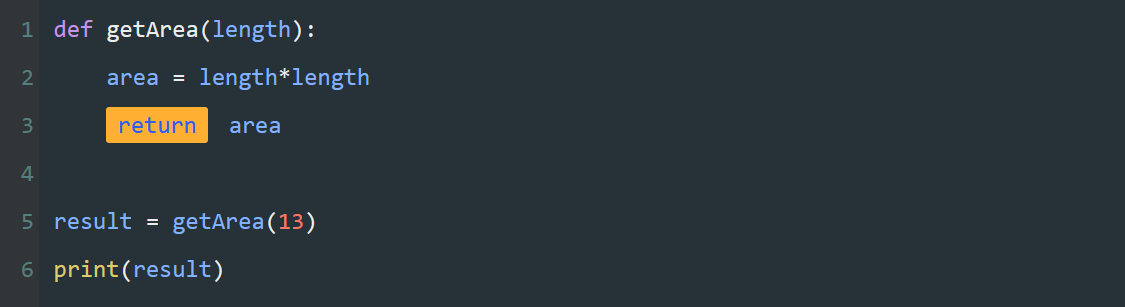return 后面是指定的返回值，返回值会返回到调用的地方。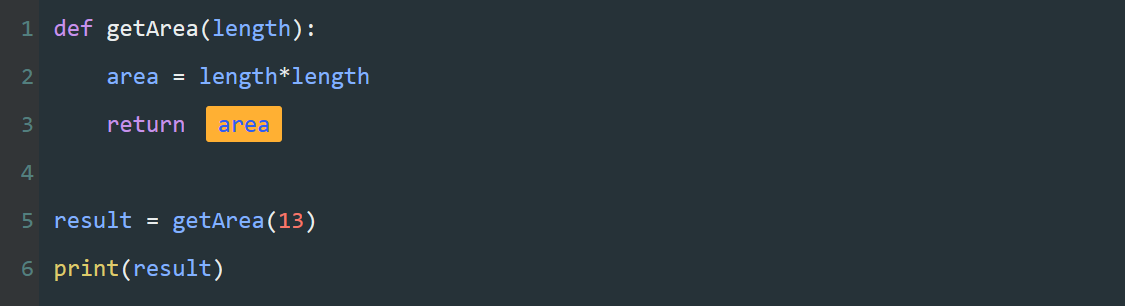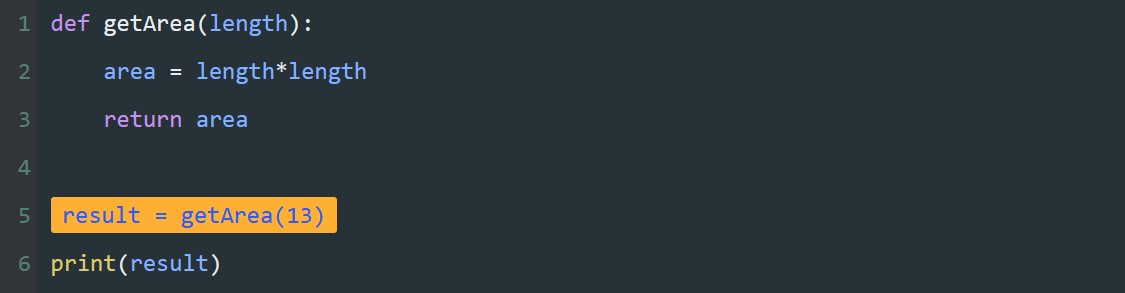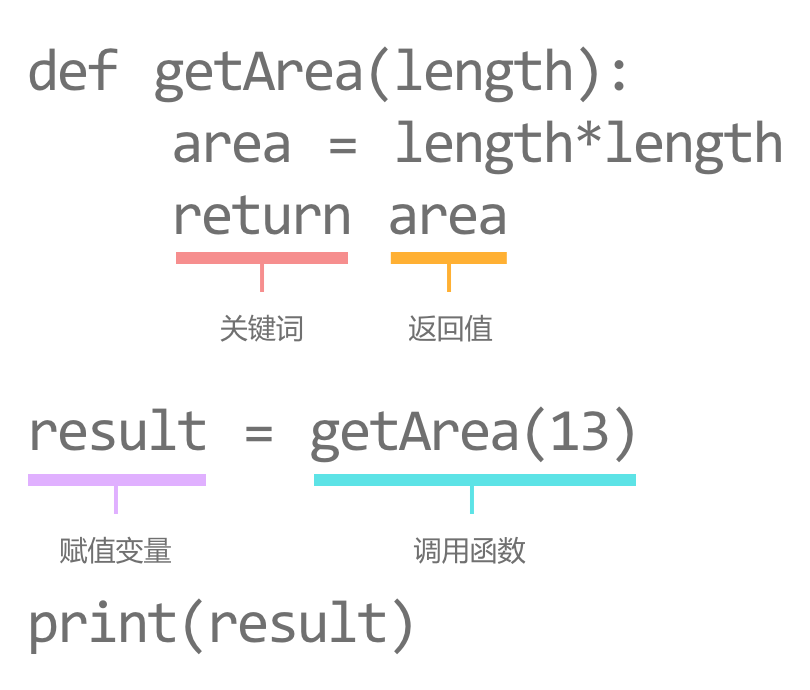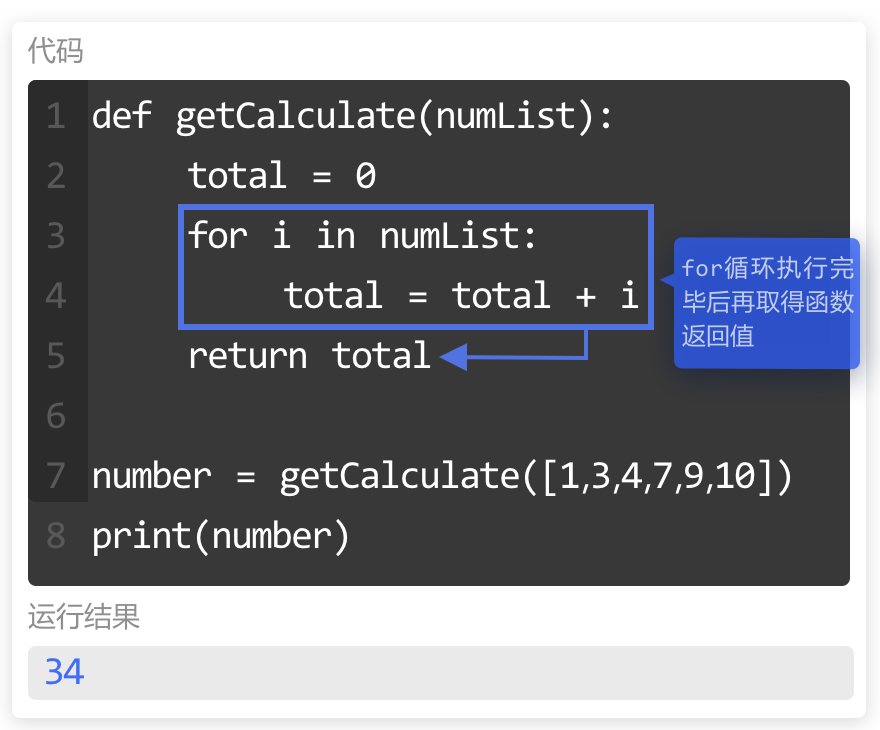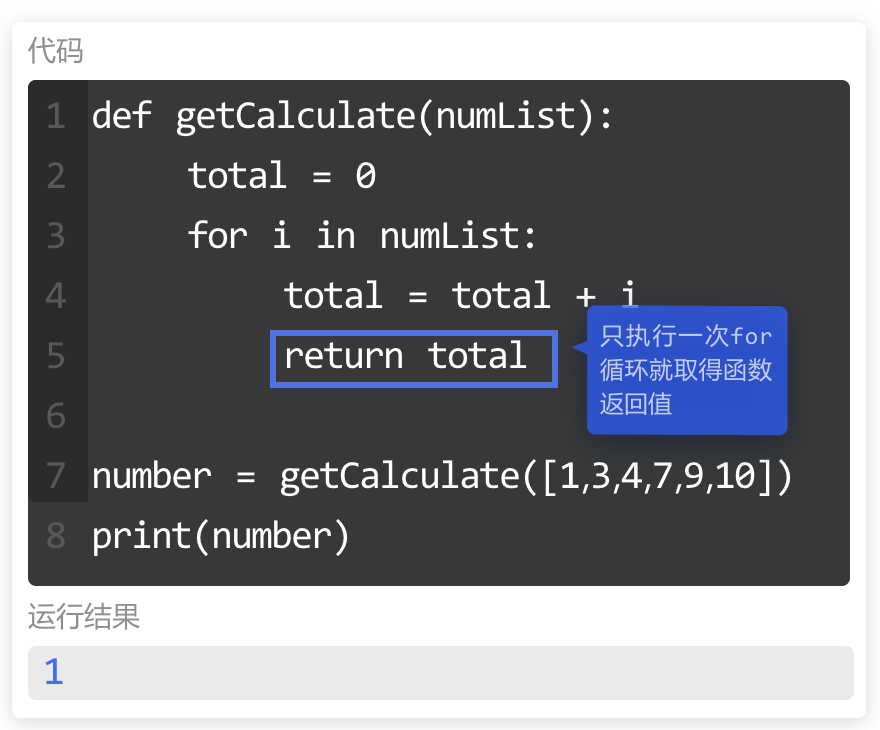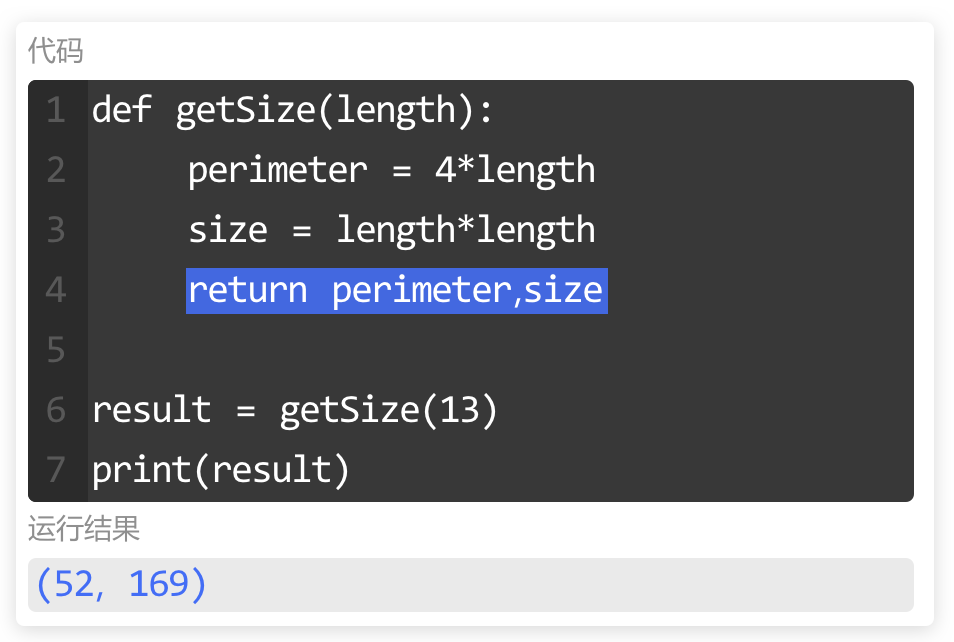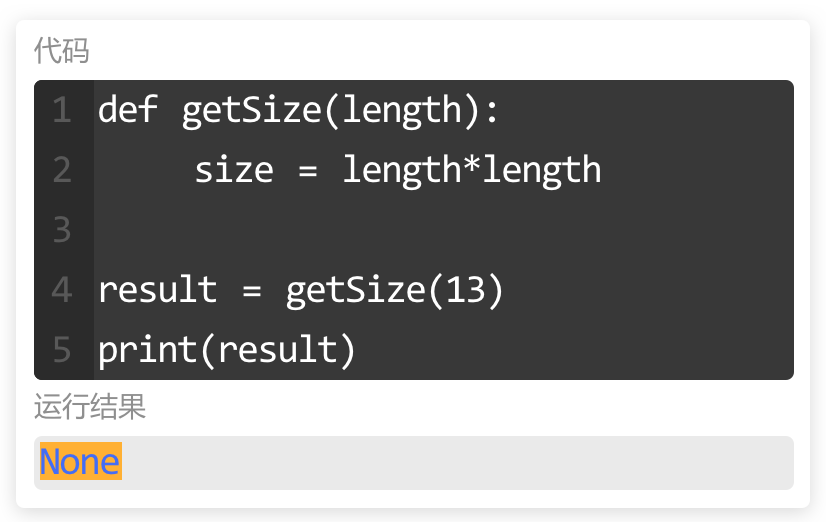### 小练习

``````def getSize(length):
perimeter = 4*length
area = length*length
return perimeter,area

result = getSize(6)
print(result)
result = getSize(8)
print(result)``````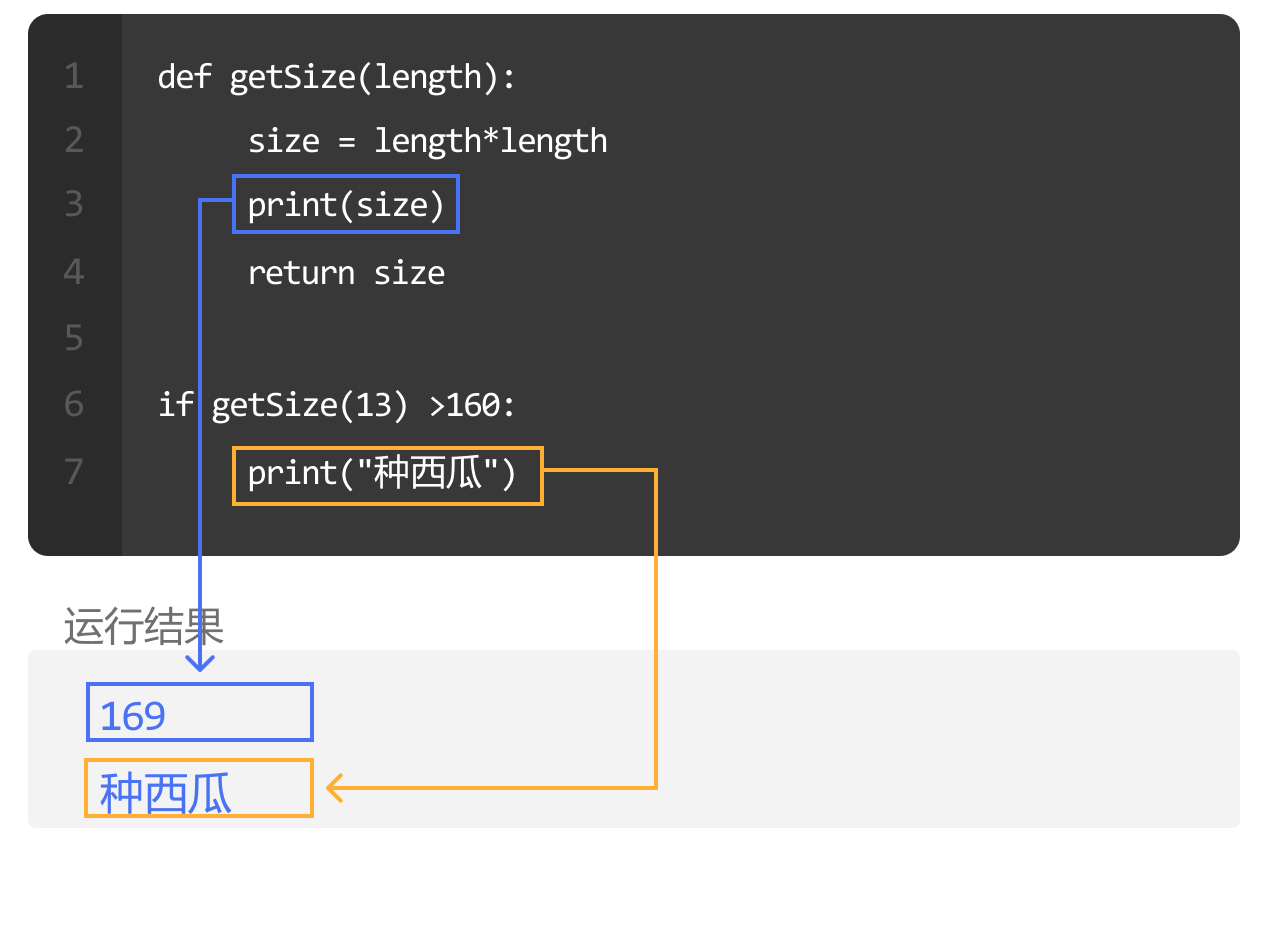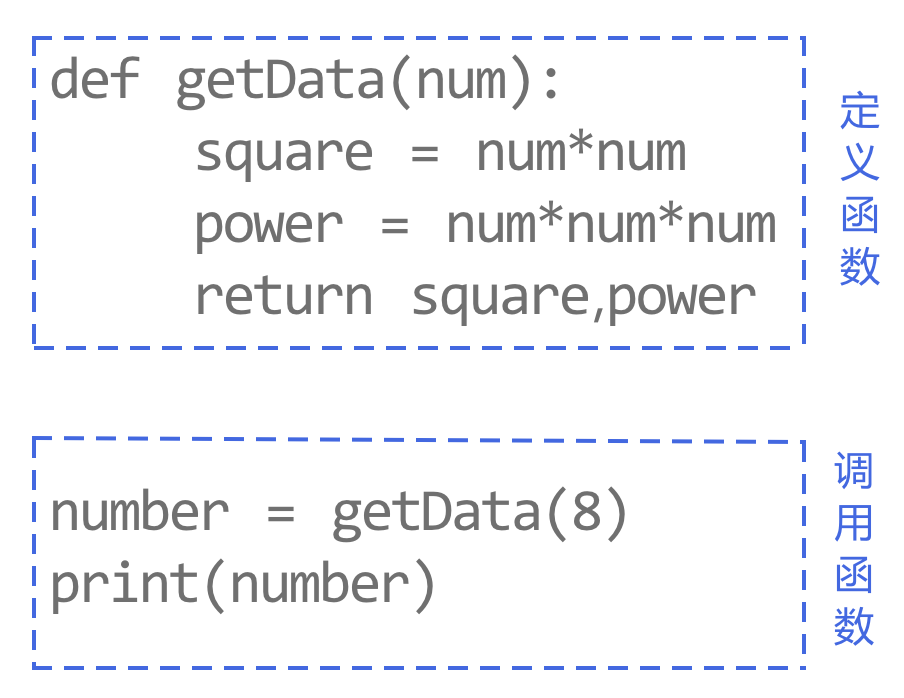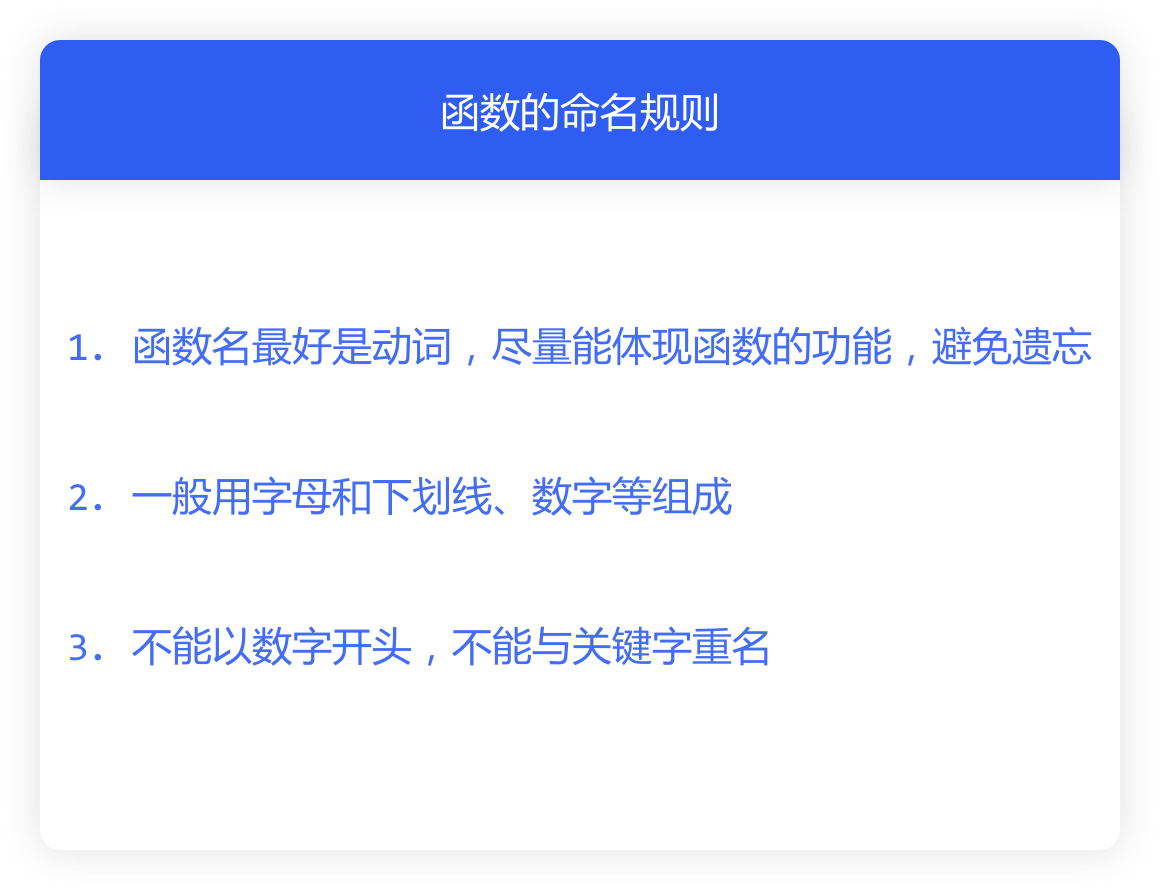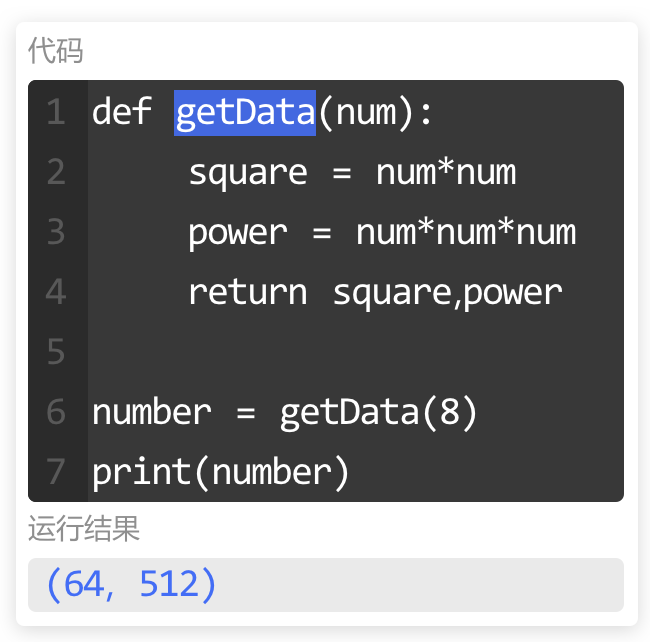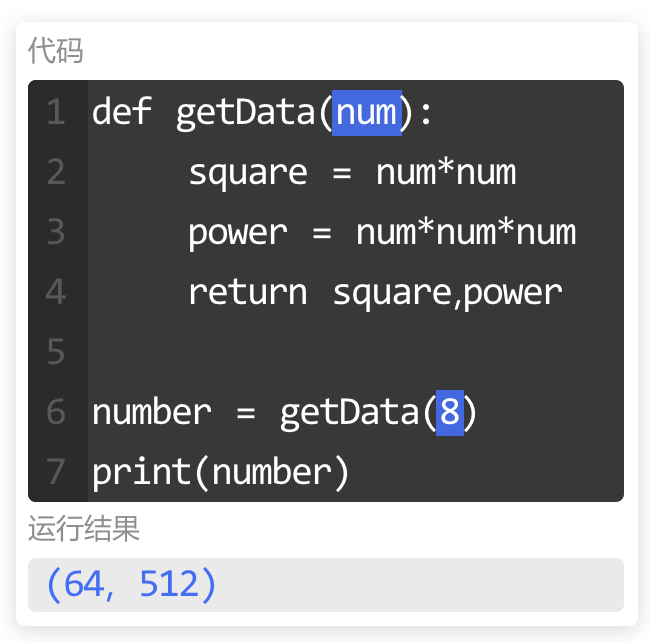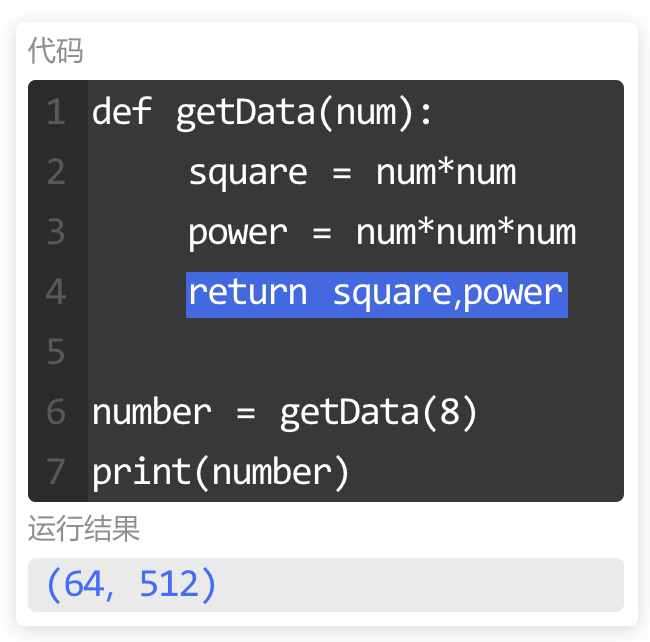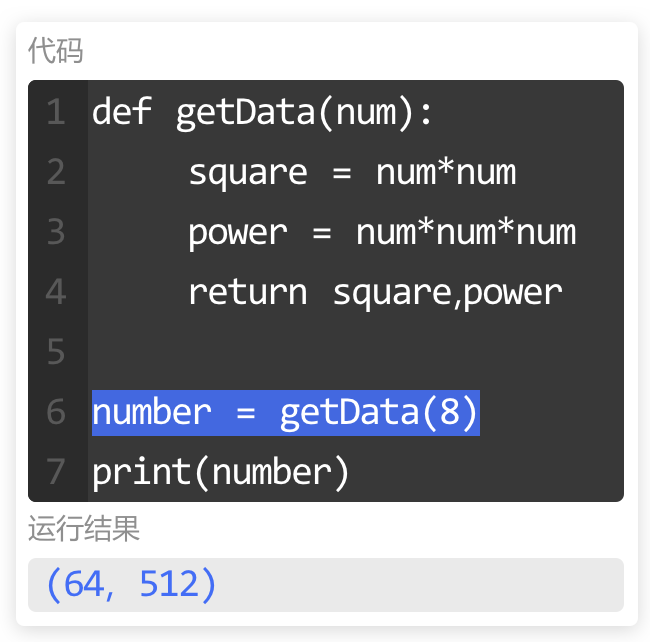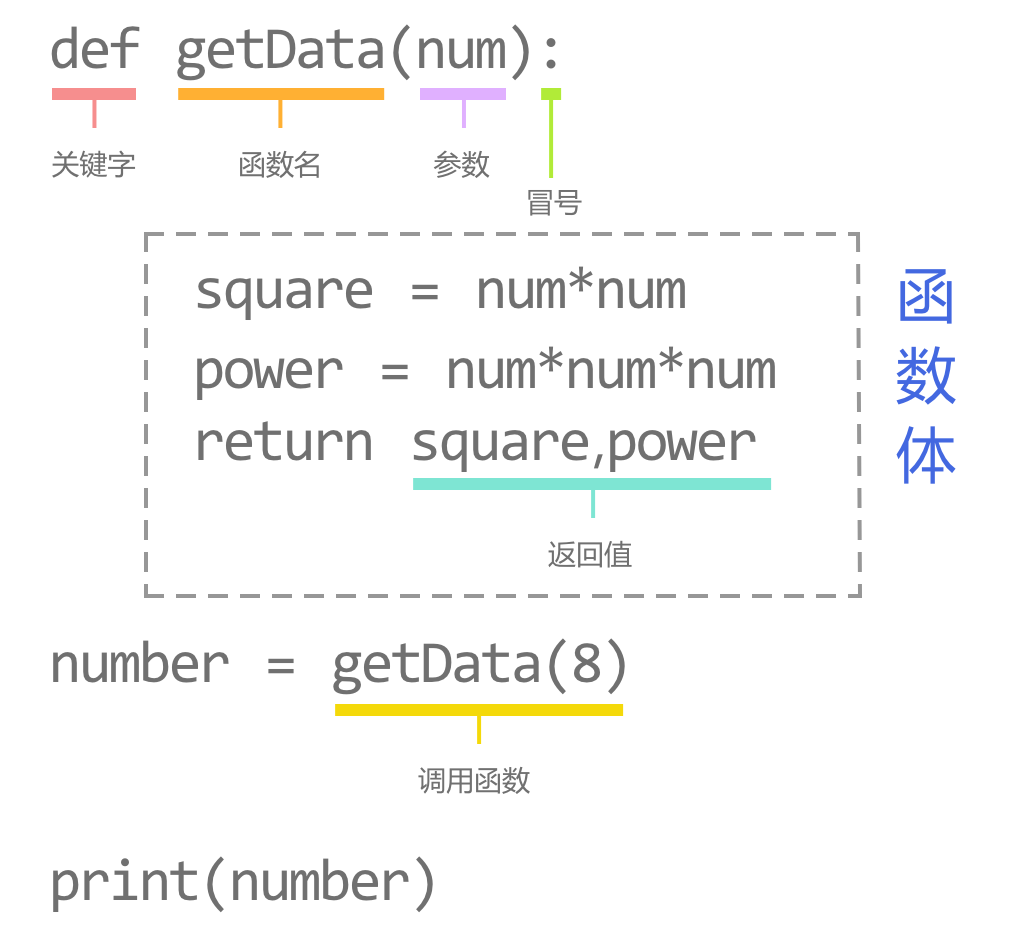## 内置函数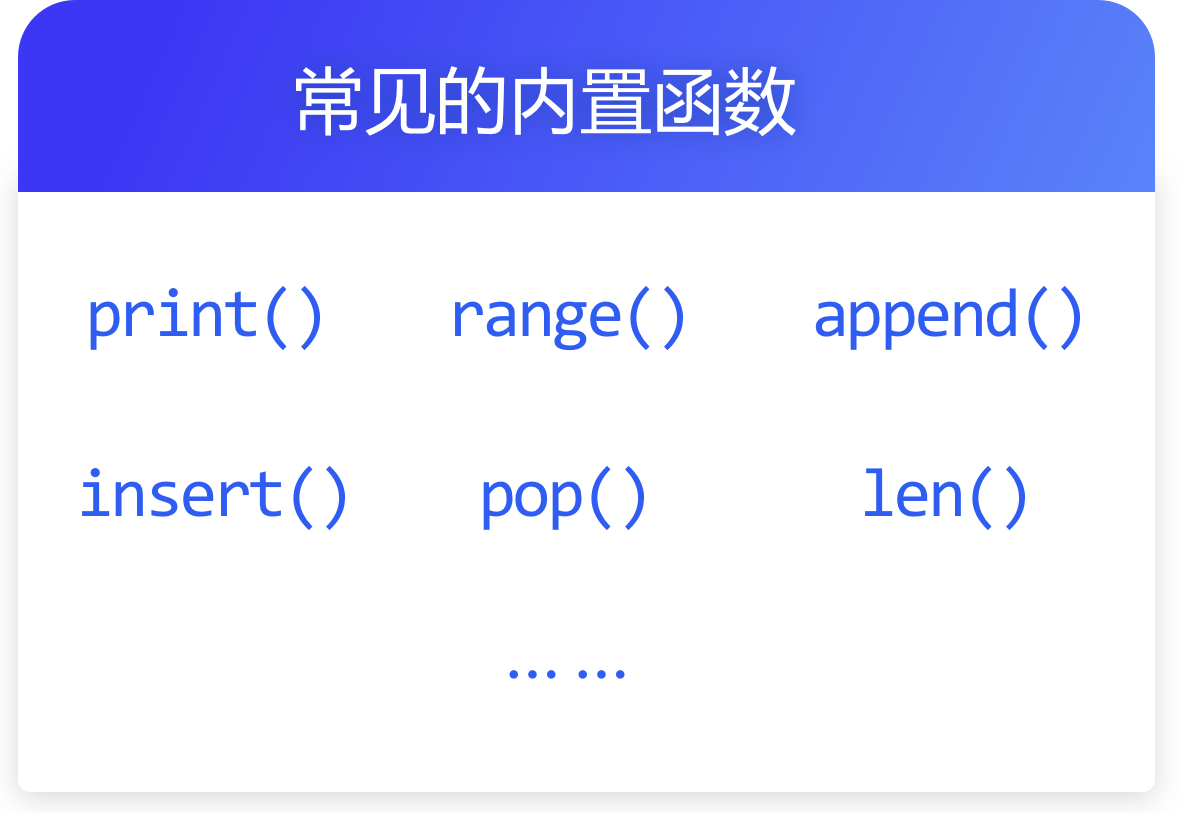`len` 为函数名；
`() `为调用符号，在此处执行该函数中的代码；

• 1人Love
• 0人Haha
• 0人Wow
• 0人Sad
• 0人Angry
Python、函数